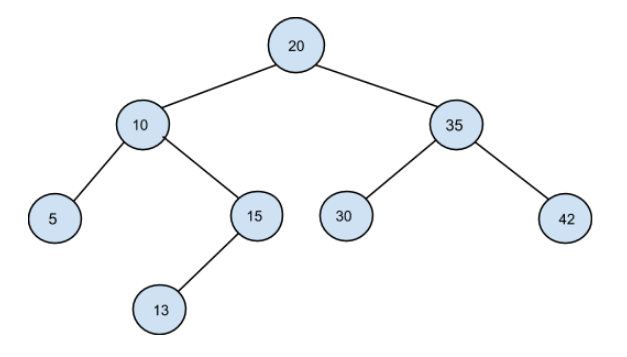# Closest Binary Search Tree Value

Posted: 2 Dec, 2020
Difficulty: Easy

## PROBLEM STATEMENT

#### A binary search tree (BST) is a binary tree data structure with the following properties.

``````• The left subtree of a node contains only nodes with data less than the node’s data.

• The right subtree of a node contains only nodes with data greater than the node’s data.

• Both the left and right subtrees must also be binary search trees.
``````
##### For Example:``````For the given BST and target value ‘K’ =  32, the closest element is 30 as the absolute difference between 30 and 32 (|32 - 30|) is the minimum among all other possible node-target pairs.
``````
##### Input Format:
``````The first line contains an Integer 'T' which denotes the number of test cases or queries to be run. Then the test cases follow.

The first line of each test case contains the elements of the tree in the level order form separated by a single space.
If any node does not have a left or right child, take -1 in its place. Refer to the example below.

The second line of each test case contains a single non-negative integer ‘K’ denoting the target value.
``````
##### Example:
``````Elements are in the level order form. The input consists of values of nodes separated by a single space in a single line. In case a node is null, we take -1 in its place.

The input for the tree depicted in the below image would be :
````````````1
2 3
4 -1 5 6
-1 7 -1 -1 -1 -1
-1 -1

Explanation :

Level 1 :
The root node of the tree is 1

Level 2 :
Left child of 1 = 2
Right child of 1 = 3

Level 3 :
Left child of 2 = 4
Right child of 2 = null (-1)
Left child of 3 = 5
Right child of 3 = 6

Level 4 :
Left child of 4 = null (-1)
Right child of 4 = 7
Left child of 5 = null (-1)
Right child of 5 = null (-1)
Left child of 6 = null (-1)
Right child of 6 = null (-1)

Level 5 :
Left child of 7 = null (-1)
Right child of 7 = null (-1)

The first not-null node (of the previous level) is treated as the parent of the first two nodes of the current level. The second not-null node (of the previous level) is treated as the parent node for the next two nodes of the current level and so on.

The input ends when all nodes at the last level are null (-1).
``````
##### Note:
``````The above format was just to provide clarity on how the input is formed for a given tree.

The sequence will be put together in a single line separated by a single space. Hence, for the above-depicted tree, the input will be given as:

1 2 3 4 -1 5 6 -1 7 -1 -1 -1 -1 -1 -1
``````
##### Output Format :
``````For each test case, print a single integer representing the closest element to the given target ‘K’.

Output for every test case will be printed in a separate line.
``````
##### Note:
``````You are not required to print the output, it has already been taken care of. Just implement the function.
``````
##### Constraints :
``````1 <= T <= 100
1 <= N <= 5 * 10^3
0 <= Node.data <= 10^5
0 <= K <= 10^5

Time Limit: 1 sec
``````Approach 1

In this approach, we will visit all the nodes in pre-order fashion( we can visit nodes in post-order or in-order fashion too) of the BST and find the absolute difference between target ‘K’ and node value. At last, we will return the maximum absolute difference.

The steps are as follows:

• In the “helper” function
• If ‘NODE’ is null, then return.
• Declare a variable ‘CURR_DIFF’ of type integer to store the absolute value of the difference of target ‘K’ and ‘NODE’ value.
• Initialize a ‘CURR_DIFF’ as abs(‘K’ - ‘NODE’ -> data ).
• If ‘CURR_DIFF’ is less than ‘MIN_DIFF,’ then update ‘MIN_DIFF’ as ‘CURR_DIFF’ and ‘CLOSEST’ as ‘NODE’ -> data.
• Else if ‘CURR_DIFF’ is equal to ‘MIN_DIFF’ then, ‘CLOSEST’ will be a minimum of ‘CLOSEST’ and ‘NODE’ -> data.
• Recursively visit the left and right subtree.

• In the given function
• Declare variables ‘MIN_DIFF’ and ‘CLOSEST’ of type integer.
• Set ‘MIN_DIFF’ initially as INT_MAX.
• Call the “helper” function for the given node, target value ‘K’, ‘MIN_DIFF’, and ‘CLOSEST’.
• Return ‘CLOSEST’.